# Measuring an angle with the protractor Online Quiz

Following quiz provides Multiple Choice Questions (MCQs) related to Measuring an angle with the protractor. You will have to read all the given answers and click over the correct answer. If you are not sure about the answer then you can check the answer using Show Answer button. You can use Next Quiz button to check new set of questions in the quiz.Q 1 - Measure the following angle using a protractor.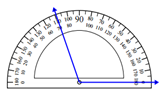### Explanation

Step 1:

We line up one ray with 0°. We then read the angle measurement where the other ray crosses the protractor.

Step 2:

Reading from the protractor, the given angle measures 110°.

Q 2 - Measure the following angle using a protractor.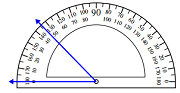### Explanation

Step 1:

We line up one ray with 0°. We then read the angle measurement where the other ray crosses the protractor.

Step 2:

Reading from the protractor, the given angle measures 45°.

Q 3 - Measure the following angle using a protractor.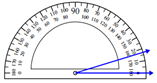### Explanation

Step 1:

We line up one ray with 0°. We then read the angle measurement where the other ray crosses the protractor.

Step 2:

Reading from the protractor, the given angle measures 17°.

Q 4 - Measure the following angle using a protractor.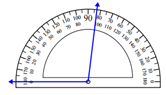### Explanation

Step 1:

We line up one ray with 0°. We then read the angle measurement where the other ray crosses the protractor.

Step 2:

Reading from the protractor, the given angle measures 97°.

Q 5 - Measure the following angle using a protractor.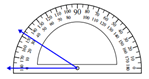### Explanation

Step 1:

We line up one ray with 0°. We then read the angle measurement where the other ray crosses the protractor.

Step 2:

Reading from the protractor, the given angle measures 32.5°.

Q 6 - Measure the following angle using a protractor.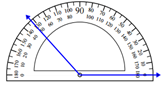### Explanation

Step 1:

We line up one ray with 0°. We then read the angle measurement where the other ray crosses the protractor.

Step 2:

Reading from the protractor, the given angle measures 132°.

Q 7 - Measure the following angle using a protractor.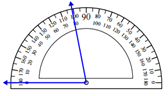### Explanation

Step 1:

We line up one ray with 0°. We then read the angle measurement where the other ray crosses the protractor.

Step 2:

Reading from the protractor, the given angle measures 100°.

Q 8 - Measure the following angle using a protractor.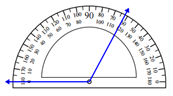### Explanation

Step 1:

We line up one ray with 0°. We then read the angle measurement where the other ray crosses the protractor.

Step 2:

Reading from the protractor, the given angle measures 118°.

Q 9 - Measure the following angle using a protractor.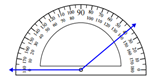### Explanation

Step 1:

We line up one ray with 0°. We then read the angle measurement where the other ray crosses the protractor.

Step 2:

Reading from the protractor, the given angle measures 140°.

Q 10 - Measure the following angle using a protractor.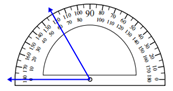### Explanation

Step 1:

We line up one ray with 0°. We then read the angle measurement where the other ray crosses the protractor.

Step 2:

Reading from the protractor, the given angle measures 60°.

measuring_angle_with_the_protractor.htm